Titolo visibileLOCALI
 Indice Ristoranti Italia Ristoranti Lazio Pizzerie Napoletane Ricerca per Regione Ricerca Avanzata Cucina Estera Cucina Regionale

PROGRAMMAZIONE
 Indice Html Flash JavaScript VBScript Java

CULTURA
 Scuole Private Aforismi Giornali Online Dizionari Matematica

# Code Comparison

## VBScript

```// This is a comment.
/* Multi-line
comment. */

```
```This is a comment.
Rem So is this.  No multi-line comments.
```
Variables, Constants, and Arrays
```
var x, y
var z = 10

No way to force variables to be declared

No constants

var Vector = new Array(10)
var Names = new Array()

var Matrix = new Array(4)
Matrix = new Array(5)

Vector = 1.5
Matrix = 2

```
```
Dim x, y
Dim z   Cant assign on same line
z = 10

Force all vars to be declared before being used.
Option Explicit

Const PI = 3.14

Dim Vector(9)    9 is last slot
 Cant do this  arrays must have upper bound

Dim Matrix(3, 4)

Vector(9) = 1.5
Matrix(1, 4) = 2

```
Output
```str = " there!"
document.write("hello" + str)
document.writeln("good\nbye")

Response.Write(str)  Server-side
Another way

```
```str = " there!"
document.write "hello" & str
document.writeln "good" & vbCr & "bye"

Response.Write str     Server-side
Another way

MsgBox "message"  Client-side
```
Functions
```
{
return a + b
}

Variable are always passed by value.
Only objects can be passed by reference.

```
```Function Add(ByVal x, ByVal y)
End Function

Sub Add2(ByVal x, ByVal y, ByRef ans)
ans = x + y
End Sub

Add2 1, 2, sum      No () for Sub params
Call Add2(1, 2, sum)    Alternate call

```
Decisions
```
if (score == 100)

if (a == 1 || a == 5)
{
vector[a] = b
c = 1
}
else if (a == 2)
b--
else
b *= 2

switch(x)
{
case 1: str = str + "end"
break
case 2:
case 3: y = y * y * y
break
default: z = z / 2
}

```
```If score = 100 Then _
MsgBox "Great!"

If a = 1 Or a = 5 Then
vector(a) = b
c = 1
ElseIf a = 2 Then
b = b - 1
Else
b = b * 2
End If

Select Case x
Case 1
str = str & "end"
Case 2, 3
y = y ^ 3
Case 4 To 10
4 <= x <= 10
Case Is >= 25
x >= 25
Case Else   Default
z = z \ 2
End Select

```
Loops
```
for (i = 1; i <= 5; i++)
document.writeln(i)

for (c = 2; c <= 10; c += 2)
a += c

for (var index in myArray)
document.write(myArray[index])

while (a < 10)
a++

do
a++;
while (a < 10)
```
```For i = 1 To 5
document.writeln i
Next

For c = 2 To 10 Step 2
a = a + c
Next

Dim element
For Each element in myArray
document.write element
Next

Do While a < 10       Do Until a = 10
a = a + 1		a = a + 1
Loop		      Loop

Do		      Do
a = a + 1		a = a + 1
Loop While a < 10     Loop Until a = 10

```
Arithmetic Operators
```
+ - * /
% (mod)
```
```
+ - * /
\  (integer division)
Mod
^  (raising to a power)
```
Relational Operators
```
<  <=  >  >=  ==  !=
```
```<  <=  >  >=  =  <>
```
Logic Operators
```
&&  ||  !

```
```And  Not  Or  Xor
Eqv  (Equivalent)
Imp
```
Bitwise Operators
```
&  ~  |  ^  <<  >>
```
```And  Not  Or  Xor  (no shift left/right)
```
Strings
```
var x
x = "HU"
x = x + " is great!"
```
```Dim x
x = "HU"
x = x & " is great!"
```
VBScript String Functions
Function Description Example
StrConv Used primarily to change the case of letters in the string. Can also do UNICODE and other format changes. x = StrConv("Test", vbUpperCase) --> TEST
UCase, LCase Returns string converted to uppercase/lowercase. x = UCase(Test) --> TEST
Len Returns the string length. length = Len(a) + Len(x) --> 1 + 4
LSet, RSet Justifies chars in fixed-length string to left or right side. RSet y = right -->  right
Left, Right Returns the specified number of chars from left-hand or right-hand side of string. x = Left(Harding, 4) --> Hard
Mid Returns substring from a search string. x = Mid(I love you., 3, 4) --> love
LTrim, RTrim, Trim Returns a copy of the string with leading, trailing, or both leading and trailing spaces removed. x = LTrim(  <-trim->  ) --><-trim->  
x = Trim(  <-trim->  ) --> <-trim->
StrComp Compares 2 strings. Returns 1 if str1 < str2, 1 if str1 > str2, 0 if strings are equal. r = StrComp(HU, ACU) --> 1
InStr Searches a string for a substring and returns the integer position if found, 0 if not found. r = InStr(1, To be or not to be, be) --> 4
Asc, AscW Asc returns ASCII value of given string. AscW returns UNICODE value. n = Asc(A) --> 65
n = AscW(A) --> 65
Chr Returns string equivalent of given ASCII value. c = Chr(65) --> A
Str Converts a number to a string. x = Str(459) --> " 459"
Split Returns an array of substrings produced from splitting a string based on a delimeter. myArray = Split("IxLovexVBScript!", "x")
myArray(0) --> I
myArray(1) --> Love
myArray(2) --> VBScript!
StrReverse Returns a reversed string. x = StrReverse("VBScript") --> "tpircSBV"

LOCALI - RICERCHE
 Avanzata Per Regione Solo Pizzerie Solo Internazionale Solo Italiana Per Tipo Cucina Per Città nel Lazio

SITO
 Contattaci Segnala localeFeed RssSegnala un errore Guestbook News Aggiungi a preferiti Imposta Homepage Segnala un Sito Iscriviti Login

RICETTE CUCINA
 Indice Ricette Primi Carne Pollame Pesce Dolci Cocktail

Questo sito e' un sito non commerciale e potrebbe riportare informazioni non aggiornate e/o non corrette. Leggi il disclaimer sottostante.

Lavoro |  Home |  Locali |  Info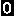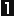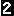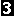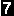Disclaimer |  Portale |  Blog |  Ricette